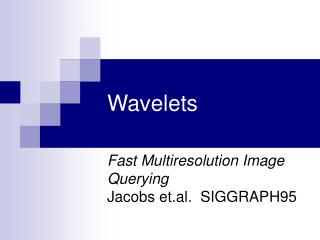# Wavelets - PowerPoint PPT PresentationDownload PresentationWavelets

Wavelets
Download Presentation## Wavelets

- - - - - - - - - - - - - - - - - - - - - - - - - - - E N D - - - - - - - - - - - - - - - - - - - - - - - - - - -
##### Presentation Transcript

1. Wavelets Fast Multiresolution Image QueryingJacobs et.al. SIGGRAPH95

2. Outline • Overview / Background • Wavelets • 2D Image matching • L1, L2 metrics • Wavelet metric • Evaluation • Use in 3D

3. Image matching • 2D analogue of 3D shape matching • Raster instead of XYZ

4. What are we trying to match? • Looking for different images of the same things? • Different projections • Different colors • Looking for images that look the same? • Look for similar shapes and colors • Metric to discern like human eye

5. Wavelets • Decompose a signal into component parts • Fourier analysis: a signal can be represented as a (possibly infinite) sum of sine and cosine functions • Signal becomes a set of wavelet coefficients • Coefficients represent features of signal

6. Wavelets II • Signal can be completely reconstructed from all the coefficients • Signal can be partially reconstructed from some coefficients

7. Wavelets for 2D Images • Each color plane in image is signal • Coefficients will represent visual features in the image • Store as many coefficients as needed • Image compression (see next slide) • c.f. Statistical shape descriptors

8. Wavelet Reconstruction SIGGRAPH 96 Course Notes: Wavelets in Computer Graphics

9. Comparing Images • Develop a metric that describes how closely two images match • Smaller difference in metric = images more similar

10. Image metrics • Comparing images Q and T, with dimensions i,j • L1-Norm • For each pixel in Q, calculate the difference between Q[i,j] and T[i,j] • Add absolute value of differences of all i,j to form metric

11. Image metrics II • L2-Norm • For each pixel in Q, calculate the square of the difference between Q[i,j] and T[i,j] • Add for all i,j, and take square root • Better than L1?

12. Image metrics III • Problems with L1 and L2 • Expensive to compute / compare: O(i*j) • Not discriminating in cases with • Color Shift • Misregistration • Noise / Dithering • In general, not good descriptors • c.f. D1, D2 in 3D

13. Wavelets as image metrics • Capture features of images e.g. edges in coefficients • Use Haar wavelets • Square basis functions • Easy to implement and compute • Calculate coefficients, truncate, quantize

14. Truncation • 128x128 image has 1282 coefficients • Truncation = only storing largest ‘n’ coefficients • ‘n’ ~ 40-60 depending on exact use • Discarding smaller coefficients discards high frequency information i.e. detail • Loss of that information is desirable

15. Quantization • Reduce precision of wavelet magnitude • Large +ve  +1 • Large –ve  -1 • Else  0 • Turns out this works well for matching

16. Wavelet metric • Q,T are query and target image coefficients • w is weighting function

17. Weighting function • Weighting function applied to give particular pairs of coefficients different significance in comparison • Gives ability to statistically tune the metric • Determined experimentally from dataset (Appendix A) • Weights expensive to calculate • Compute fewer • Bins to map range of i,j onto a weight

18. Wavelet metric II • For i=0, j=0 value in Q and T is proportional to the average overall color • From quantization, use ≠

19. Calculating coefficients • Standard two-dimensional Haar wavelet decomposition • Decompose each row, then decompose each column of the result • Trivial to implement

20. Wavelet metric III • Final metric is • T[0,0] • Sign, i and j of n largest coefficients in T

21. Faster Matching • To speed up matching, use 6 arrays • One for each combination of R,G,B,+,- • DR+,DR-, … • Each i,j in Dx is a list of all images with a metric coefficient in that color range, with that sign

22. Evaluation • Better than L1 and L2 • Matches to ~1% of database • Compact • Fast to compare: similar complexity to an 8x8 pixel image L1/L2 for any resolution • More robust • Misregistration • Color shifting • Dithering • Different resolutions

23. Evaluation II

24. Evaluation III • Limits • Scaling ~1.5 times • Rotation ~20 degrees • Translation ~ 15% of width

25. Matching in 3D • Can this be used in 3D as well • Compares image rather than geometry • Render 3D into voxels? • Projection of 3D object into 2D? • In general, other 3D specific methods probably much better